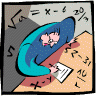## Mathematics, Department of## Dissertations, Theses, and Student Research Papers in Mathematics

#### Date of this Version

5-2015

A DISSERTATION Presented to the Faculty of The Graduate College at the University of Nebraska In Partial Fulfillment of Requirements For the Degree of Doctor of Philosophy, Major: Mathematics, Under the Supervision of Professor Jamie Radcliffe. Lincoln, Nebraska: May, 2015

#### Abstract

This dissertation answers several questions in extremal graph theory, each concerning the maximum or minimum number of certain substructures a graph can have, given that it must satisfy certain properties. In recent years there has been increased interest in such problems, which are extremal problems for "counting" parameters of graphs. The results in this dissertation focus on graphs that have n vertices and e edges and 3-uniform hypergraphs that have n vertices and e edges.

We first observe in the preliminaries chapter that for graphs with a fixed number of vertices and edges there is a threshold graph attaining the minimum number of matchings. The first two major results develop this fact in two different directions. In Chapter 3 we consider the problem of maximizing the number of matchings in the class of threshold graphs. We solve the problem completely, concluding that a graph in this class has the maximum number of matchings if and only if it is almost alternating. The second and more fundamental question is the problem of which threshold graph, and hence which graph, has the minimum number of matchings. Ahlswede and Katona determined which graph has the fewest matchings of size 2. In Chapter 4 we extend this result to all sizes of matchings and to the total number of matchings. We prove that either the lex graph or the colex graph minimizes the number of matchings. We further prove that the lex bipartite graph has the fewest matchings among all bipartite graphs with parts of fixed sizes.

Finally, in Chapter 5, we answer an extremal question about independent sets in hypergraphs. ... The final chapter discusses results about maximizing s-independent sets in r-uniform hypergraphs, the most significant result finds the 3-uniform hypergraph maximizing the number of 2-independent sets for certain numbers of vertices and edges.